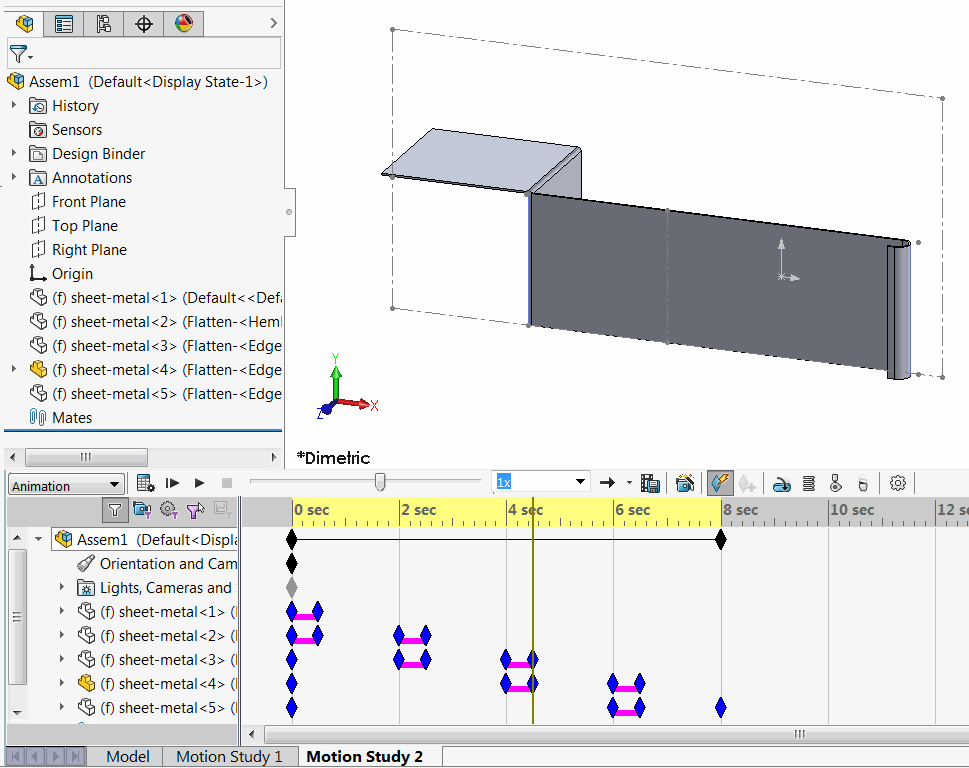More 'Goodies'

Macro demonstrates how to create an animation from configurations using SOLIDWORKS API.

This could be useful when it is required to create an animation to represents model history or sheet metal folding.

• Open part or assembly
• Select configurations in the order they should be animatedMultiple configurations selected in the configurations tab
• Run the macro. New assembly created with configurations set as animation steps.Sheet metal bending animation

Macro parameters (time of the bend transition and pause between folding operations) can be changed by modifying the constants at the top of the macro

``````Const TRANSITION_TIME As Double = 0.5
Const PAUSE_TIME As Double = 2
``````

Refer the Suppress Features In New Configurations for a macro to create configurations from features.

```Const TRANSITION_TIME As Double = 0.5
Const PAUSE_TIME As Double = 2

Dim swApp As SldWorks.SldWorks

Sub main()

Set swApp = Application.SldWorks

Dim swModel As SldWorks.ModelDoc2

Set swModel = swApp.ActiveDoc

If Not swModel Is Nothing Then

If swModel.GetPathName() <> "" Then

Dim vConfs As Variant
vConfs = GetSelectedConfigurations(swModel)

If Not IsEmpty(vConfs) Then

Dim swAssy As SldWorks.AssemblyDoc

Set swAssy = NewAssembly

If Not swAssy Is Nothing Then
Dim vComps As Variant
vComps = CreateComponents(swAssy, swModel, vConfs)
Dim swMotionStudyMgr As Object
Set swMotionStudyMgr = swAssy.Extension.GetMotionStudyManager()

Dim swMotionStudy As Object
Set swMotionStudy = swMotionStudyMgr.CreateMotionStudy()

CreateFrames swMotionStudy, vComps, TRANSITION_TIME, PAUSE_TIME
Else

MsgBox "Failed to create new assembly"
End If
Else
MsgBox "Please select configurations"
End If

Else
MsgBox "Please save document"
End If

Else
MsgBox "Please open part or assembly"
End If

End Sub

Sub CreateFrames(motionStudy As Object, vComps As Variant, transitionTime As Double, pauseTime As Double)

Dim i As Integer
Dim swCompToHide As SldWorks.Component2
Dim swCompToShow As SldWorks.Component2

motionStudy.SetTime 0

Set swCompToShow = vComps(0)
swCompToShow.Visible = True

For i = 1 To UBound(vComps)
Set swCompToHide = vComps(i)
swCompToHide.Visible = False
Next

Dim curTime As Double
curTime = 0

For i = 1 To UBound(vComps)

Set swCompToHide = vComps(i - 1)
Set swCompToShow = vComps(i)

motionStudy.SetTime curTime + transitionTime
swCompToHide.Visible = False

motionStudy.SetTime curTime + transitionTime
swCompToShow.Visible = True

curTime = i * showTime + i * pauseTime
motionStudy.SetTime curTime

swCompToShow.Visible = False
swCompToShow.Visible = True

If i <> UBound(vComps) Then

Dim swCompToLock As SldWorks.Component2
Set swCompToLock = vComps(i + 1)

swCompToLock.Visible = True
swCompToLock.Visible = False

End If

Next

End Sub

Function CreateComponents(assy As SldWorks.AssemblyDoc, model As SldWorks.ModelDoc2, confs As Variant) As Variant

Dim i As Integer

Dim swComps() As SldWorks.Component2
ReDim swComps(UBound(confs))

Dim dMatrix(15) As Double
dMatrix(0) = 1: dMatrix(1) = 0: dMatrix(2) = 0: dMatrix(3) = 0
dMatrix(4) = 1: dMatrix(5) = 0: dMatrix(6) = 0: dMatrix(7) = 0
dMatrix(8) = 1: dMatrix(9) = 0: dMatrix(10) = 0: dMatrix(11) = 0
dMatrix(12) = 1: dMatrix(13) = 0: dMatrix(14) = 0: dMatrix(15) = 0

Dim swMathUtils As SldWorks.MathUtility
Set swMathUtils = swApp.GetMathUtility
Dim swTransform As SldWorks.MathTransform
Set swTransform = swMathUtils.CreateTransform(dMatrix)

For i = 0 To UBound(confs)

Dim swComp As SldWorks.Component2
Set swComp = assy.AddComponent5(model.GetPathName(), swAddComponentConfigOptions_e.swAddComponentConfigOptions_CurrentSelectedConfig, "", True, confs(i), 0, 0, 0)
swComp.Select4 False, Nothing, False
assy.UnfixComponent
swComp.Transform2 = swTransform
swComp.ReferencedConfiguration = confs(i)
swComp.Select4 False, Nothing, False
assy.FixComponent
Set swComps(i) = swComp
Next

CreateComponents = swComps

End Function

Function NewAssembly() As SldWorks.AssemblyDoc

Dim swAssy As SldWorks.AssemblyDoc

Dim assyTemplate As String
assyTemplate = swApp.GetUserPreferenceStringValue(swUserPreferenceStringValue_e.swDefaultTemplateAssembly)

If assyTemplate <> "" Then
Set swAssy = swApp.NewDocument(assyTemplate, 0, 0, 0)
Else
Err.Raise vbObjectError, , "Assembly default template is not specified"
End If

Set NewAssembly = swAssy

End Function

Function GetSelectedConfigurations(model As SldWorks.ModelDoc2) As Variant

Dim confNames() As String
Dim isInit As Boolean

Dim swSelMgr As SldWorks.SelectionMgr
Set swSelMgr = model.SelectionManager

Dim i As Integer

For i = 1 To swSelMgr.GetSelectedObjectCount2(-1)

Dim swConf As SldWorks.Configuration
On Error Resume Next

Set swConf = swSelMgr.GetSelectedObject6(i, -1)

If Not swConf Is Nothing Then
If True = isInit Then
ReDim Preserve confNames(UBound(confNames) + 1)
Else
isInit = True
ReDim confNames(0)
End If

confNames(UBound(confNames)) = swConf.Name

End If

Next

GetSelectedConfigurations = confNames

End Function```